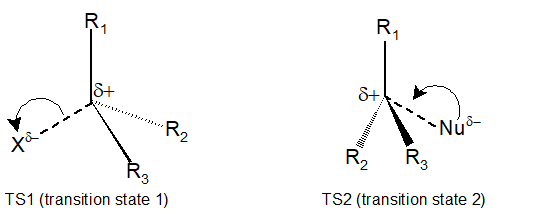# 7.8: The Sₙ1 Reaction

Learning Objective

• determine the rate law & predict the mechanism based on its rate equation or reaction data for SN1 reactions
• predict the products and specify the reagents for SN1 reactions with stereochemistry
• propose mechanisms for SN1 reactions
• draw and interpret Reaction Energy Diagrams for SN1 reactions

### The SN1 mechanism with Stereochemistry

A second model for a nucleophilic substitution reaction is called the 'dissociative' or 'SN1' mechanism. In many cases, the nucleophile is the solvent, so this mechanism can also be called "solvolysis".

Step1: In the SN1 mechanism, the carbocation forms when the C-X bond breaks first, before the nucleophile approachesTh carbocation has a central carbon with only three bonds and bears a formal charge of +1. Recall that a carbocation should be pictured as sp2 hybridized, with trigonal planar geometry. Perpendicular to the plane formed by the three sp2 hybrid orbitals is an empty, unhybridized p orbital.Step 2: The nucleophile reacts with the empty, 'electron hungry' p orbital of the carbocation to form a new bond and return the carbon to tetrahedral geometry. Because of this trigonal planar geometry, the nucleophile can approach the carbocation from either lobe of the empty p orbital (aka either side of the carbocation). This means that about half the time the product has the same stereochemical configuration as the starting material (retention of configuration), and about half the time the stereochemistry has been inverted. In other words, racemization of the product occurs during SN1 reactions if the electrophilic carbon is chiral. If the intermediate from a chiral alkyl halide survives long enough to encounter a random environment, the products are expected to be racemic (a 50:50 mixture of enantiomers). On the other hand, if the departing halide anion temporarily blocks the front side, or if a nucleophile is oriented selectively at one or the other face, then the substitution might occur with predominant inversion or even retention of configuration.As an example, the tertiary alkyl bromide below, (S)-3-bromo-3-methylhexane, would be expected to form a racemic mix of R- and S-3-methyl-3-hexanol after an SN1 reaction with water as the nucleophile.Exercise

1. Draw the structure of the intermediate in the two-step nucleophilic substitution reaction (SN1) above.

Solution

1.## The SN1 Reaction Energy Diagram

The SN1 reaction is an example of a two-step reaction with a reaction intermediate. Evaluating reactive intermediates is a very important skill in the study of organic reaction mechanisms. Many important organic reactions do not occur in a single step; rather, they are the sum of two or more discreet bond-forming / bond-breaking steps, and involve transient intermediate species that go on to react very quickly. In the SN1 reaction, the carbocation species is a reaction intermediate. A potential energy diagram for an SN1 reaction shows that the carbocation intermediate can be visualized as a kind of valley in the path of the reaction, higher in energy than both the reactant and product but lower in energy than the two transition states.Exercise

2. Draw structures representing transition state 1 (TS1) and transition state 2 (TS2) in the reaction above. Use the solid/dash wedge convention to show three dimensions.

Solution

2.## SN1 Reaction Kinetics

In the first step of an SN1 mechanism, two charged species are formed from a neutral molecule. This step is much the slower of the two steps, and is therefore rate-determining. In the reaction energy diagram, the activation energy for the first step is higher than that for the second step indicating that the SN1 reaction has first order kinetics because the rate determining step involves one molecule splitting apart, not two molecules colliding. It is important to remember that first order refers to the rate law expression where the generic term substrate is used to describe the alkyl halide.

rate = k [substrate]

Because an SN1 reaction is first order overall the concentration of the nucleophile does not affect the rate. The implication is that the nucleophile does not participate in the rate limiting step or any prior steps, which suggests that the first step is the rate limiting step. Since the nucleophile is not involved in the rate-limiting first step, the nature of the nucleophile does not affect the rate of an SN1 reaction.Exercise

3. Consider two nucleophilic substitutions that occur uncatalyzed in solution. Assume that reaction A is SN2, and reaction B is SN1. Predict, in each case, what would happen to the rate of the reaction if the concentration of the nucleophile were doubled, while all other conditions remained constant.4. Give the products of the following SN1 reaction. Show stereochemistry.Solution

3. For Reaction A, the rate law is rate = k[CH3I][CH3S-]. Therefore, if the concentration of the nucleophile, CH3S-, is doubled and the concentration of the alkyl halide remains the same, then the reaction rate will double.

For Reaction B, the rate law is rate = k[CH3)3Br]. Therefore, if the concentration of the nucleophile, CH3SH, is doubled and the concentration of the alkyl halide remains the same, then reaction rate stays the same.

4.